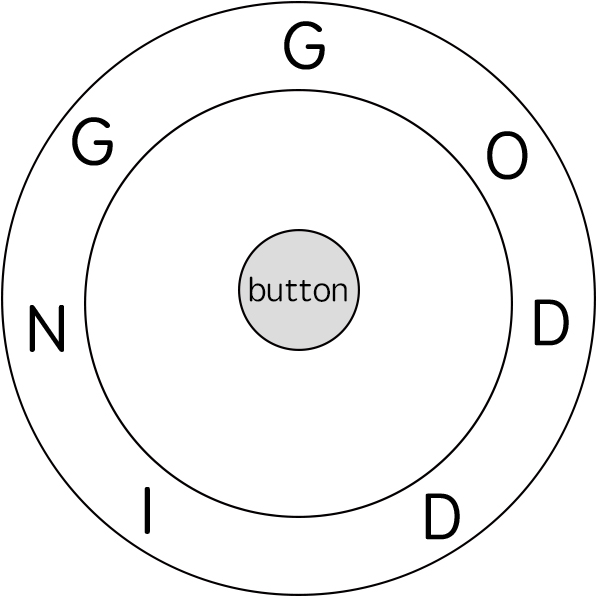## Algorithm

Problem Name: 514. Freedom Trail

In the video game Fallout 4, the quest "Road to Freedom" requires players to reach a metal dial called the "Freedom Trail Ring" and use the dial to spell a specific keyword to open the door.

Given a string `ring` that represents the code engraved on the outer ring and another string `key` that represents the keyword that needs to be spelled, return the minimum number of steps to spell all the characters in the keyword.

Initially, the first character of the ring is aligned at the `"12:00"` direction. You should spell all the characters in `key` one by one by rotating `ring` clockwise or anticlockwise to make each character of the string key aligned at the `"12:00"` direction and then by pressing the center button.

At the stage of rotating the ring to spell the key character `key[i]`:

1. You can rotate the ring clockwise or anticlockwise by one place, which counts as one step. The final purpose of the rotation is to align one of `ring`'s characters at the `"12:00"` direction, where this character must equal `key[i]`.
2. If the character `key[i]` has been aligned at the `"12:00"` direction, press the center button to spell, which also counts as one step. After the pressing, you could begin to spell the next character in the key (next stage). Otherwise, you have finished all the spelling.

Example 1:```Input: ring = "godding", key = "gd"
Output: 4
Explanation:
For the first key character 'g', since it is already in place, we just need 1 step to spell this character.
For the second key character 'd', we need to rotate the ring "godding" anticlockwise by two steps to make it become "ddinggo".
Also, we need 1 more step for spelling.
So the final output is 4.
```

Example 2:

```Input: ring = "godding", key = "godding"
Output: 13
```

Constraints:

• `1 <= ring.length, key.length <= 100`
• `ring` and `key` consist of only lower case English letters.
• It is guaranteed that `key` could always be spelled by rotating `ring.`

## Code Examples

### #1 Code Example with Javascript Programming

```Code - Javascript Programming```

``````
const findRotateSteps = function(ring, key) {
function findLeft(i, j) {
let k = i
let count = 0
while (ring[k] !== key[j]) {
k--
count++
if (k === -1) k += ring.length
}
return [k, count]
}
function findRight(i, j) {
let k = i
let count = 0
while (ring[k] !== key[j]) {
k++
count++
if (k === ring.length) k -= ring.length
}
return [k, count]
}
const dp = []
for (let i = 0; i < ring.length; i++) {
dp[i] = []
}
function f(i, j) {
if (dp[i][j] !== undefined) return dp[i][j]
if (j === key.length) return (dp[i][j] = 0)
const [i1, c1] = findLeft(i, j)
const [i2, c2] = findRight(i, j)
return (dp[i][j] = Math.min(c1 + 1 + f(i1, j + 1), c2 + 1 + f(i2, j + 1)))
}
return f(0, 0)
}
``````
Copy The Code &

Input

cmd
ring = "godding", key = "gd"

Output

cmd
4

### #2 Code Example with Python Programming

```Code - Python Programming```

``````
class Solution:
def findRotateSteps(self, ring, key):
ind, n, dp, pre = collections.defaultdict(list), len(ring),  * len(ring), key
for i, c in enumerate(ring): ind[c].append(i)
for i in ind[key]: dp[i] = min(i, n - i) + 1
for c in key[1:]:
for i in ind[c]: dp[i] = min(dp[j] + min(i - j, j + n - i) if i >= j else dp[j] + min(j - i, i + n - j) for j in ind[pre]) + 1
pre = c
return min(dp[i] for i in ind[key[-1]])
``````
Copy The Code &

Input

cmd
ring = "godding", key = "gd"

Output

cmd
4